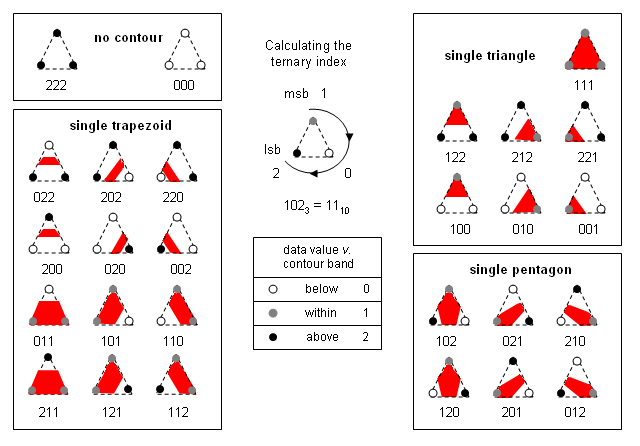# Implicit surfaces

Hi,

Is it possible to get a reference in any scripting language how to implement Meandering triangles algorithm:It is similar to 2D marching squares but with 2D triangles.

1 Like

What sort of triangles do you have? An infinite repeating pattern? A mesh? A finite region?

I have 2D flat equilateral triangle grid - one flat mesh - with iso valiues for each vertex from implicit function.

But I am wondering if it is possible to implement for irregular 2D triangular grids too?

Wikipedia says that values of triangle vertices 000 and 111 produces empty panels while other produces lines.
But the line part for coding is in question. Which tiles goes where?

In picture above codes likes 112 shows tile topology. But does it work for any kind of triangles mesh?

And I get values like this for each vertex in triangle:

4.23012 4.23012 6.75002
7.24966 5.27462 3.47486
7.24966 6.84827 5.27462
6.84827 3.54192 5.27462
6.84827 5.75856 3.54192

0.301926 -0.721459 0.368248

Do they have to be remapped between 0 and 1 or it is only positive and negative?

Ok one part I get it, that if 3 values are positive or 3 values a negative, nothing is drawn.
And this seems to correlate with plotted graph. other tiles seems to be a mistery for me but let see…

Theoretically yes. The table shows all possible triangle topologies. The Metaball algorithm in Grasshopper uses a similar approach, except it relies on squares rather than triangles. Triangles are more flexible and allow for local increased detail, but they have more complicated adjacencies.

Even given the above outline, you still have to decide whether you’re happy to evaluate all triangles and collate the results afterwards, or whether you specifically want to trace the region paths across the mesh. The latter is faster, but more difficult and risks missing certain loops.

Do note that in Rhino you already have access to a Mesh/Plane intersection algorithm, so you could simply elevate the points to the appropriate value and then slice the mesh.

Also what sort of output are you looking for? Just polylines? Individual line segments? Does it matter whether a boundary curve belongs to the below-within or the within-above transition?

I am doing it hard way in C++ application.
I am drawing a line segment per triangle and add them to collection of lines.

I am searching just for simplest and slowest approach for learning purpose.
Supposedly, looping through all triangles and collate results afterwards without any adaptive data-structures while having low skills in that evil programming language.

Nice to see that wikipedia works.

Now I have to figure out the rest of 6 tiles…:

Can’t help you with C++, but here’s about the simplest C# implementation I can come up with: boundaries.gh (11.7 KB)

It’s a bit simpler than the approach you referenced; instead of tracking both a lower and an upper threshold and creating the space in between I’m only comparing the triangle corners to a single value. If all three corners are either below or above the threshold, no shape is outputted for that triangle. If one corner is on the opposite side of the other two, then either a triangle or a quad is appended to the the output list. The benefit of this is that there’s only eight cases to take into consideration, rather than the 27 posted above.

Doing it this way at least gives you a result that could not possibly have been accomplished with the Mesh/Intersection approach I suggested as an alternative.

Nice one implementation thank you

And if you keep the below triangles in their entirety you end up with the following: boundaries2.gh (12.8 KB)

1 Like

Hehe, works for me in C++:
Where did you get the intersection function from?

I will try to implement tiles too, wikipedia says that it is only 8 case, but after implementation it does not match. I do not get it.

I thought of it. It’s just a zero-finder for a linear equation in the form `ax+b` so it’s not particularly difficult.

I was doing it right but with different if statement and I mixed tiling order. now works.
It is really simple, but linear interpolation is really nice bit.

``````	for (Surface_mesh::Vertex v : mesh.vertices(f)) {

fv[k] = mesh.position(v);
float value = v_iso[v];

//From wikipedia tiling
if (value < 0)
type += pow(10, 2-k);

++k;

}

Point p0 = (fv+fv)*0.5;
Point p1 = (fv + fv)*0.5;
Point p2 = (fv + fv)*0.5;

switch (type)
{
case(11):
case(100):
segment_points.push_back(p0);
segment_points.push_back(p2);
break;
case(101):
case(10):
segment_points.push_back(p0);
segment_points.push_back(p1);
break;
case(110):
case(1):
segment_points.push_back(p1);
segment_points.push_back(p2);
break;

default:
break;
}``````

Hello Petras
I have done a multiple iso splitting of mesh. It didn’t use exactly this algorithm. Hope it could help.

multiple_iso_splitting_mesh_LEGACY.gh (17.9 KB)